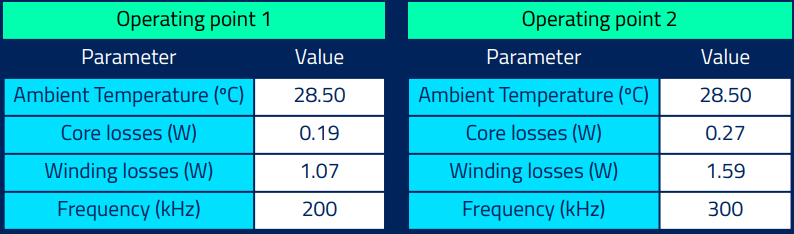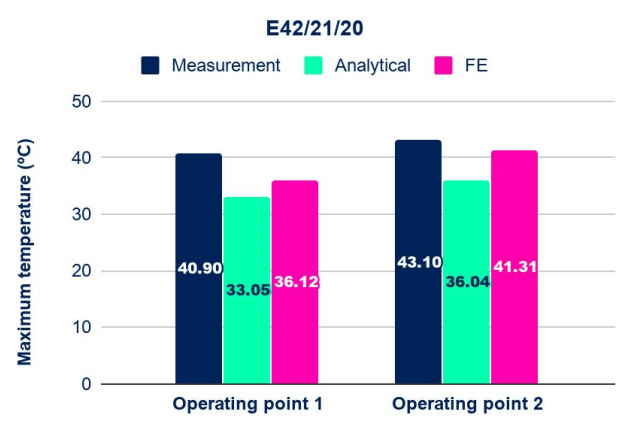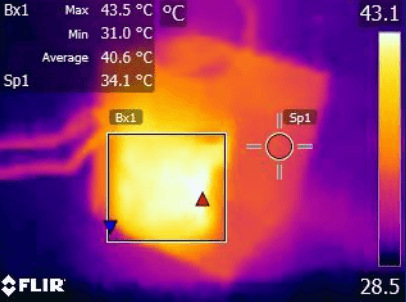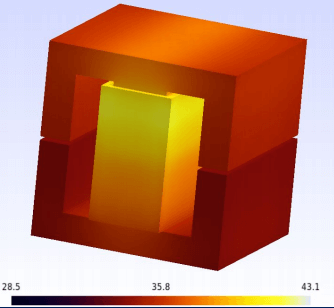# Temperature Model Using Finite Element Method

One of the parameters to be taken into account in the high-frequency magnetic components design process is the temperature rise.  Power density, loss distribution, materials used in the manufacturing process and dimensions are implicated directly in thermal performance. High temperatures affect the magnetic components, changing its performance, and also it can cause damage, even breakdown.

The objective of this application note is to compare a conventional analytical thermal model frequently used for the thermal analysis, with thermal finite element analysis, and contrast the results with real measurements.

## Thermal models

The analytical temperature and losses models used in this document are the same as used in "Magnetic transformer temperature model" application note, based on Steinmetz and Dowell's equations.

In the finite element analysis was performed a complete steady-state 3D model of the magnetic component is performed, taking into account the material thermal conductivity and the convective coefficient, neglecting radiation effects. The ambient temperature influence is also taken into account in the model, making an air environment at a certain room temperature surrounding the core.

### Results

The analysis, simulations, and measurements were done using the following transformer, under differents operating points:

• Core shape: E42/21/20
• Stacks: 2
• Core material: Ferroxcube 4S60
• Wiring: Litz 270x0.05 / Litz 105x0.05
• Number of turns: 3 / 16
• Number of parallels: 6 / 3
• Gap: 0.8 mmThe graph above shows the maximum temperature measurements and the temperature estimations with analytical and finite element (FE) methods over the two different operating points. On the other hand, the figures below show the transformer's real temperature and the result of the finite element simulation for operating point two.### Conclusions

This application note compares different methods for predicting the maximum temperature reached in the operation of high-frequency magnetic elements. To do this, two different prediction methods are compared; the finite element method provides an error below 11.7% compared with the laboratory measurements in the worst case, and 4% using the best case. With the analytical method, the error reaches 19.2% in the worst case.

Focusing on the analysis time of each method, the analytical method is fast and useful to provide a first approximation of the temperature rise in a magnetic component under certain conditions. The finite element method can be used to obtain greater precision and determine where the hot-spot areas are. The combination of these two methods allows us to reduce the cost and design time, providing a good estimation without real measurements.